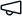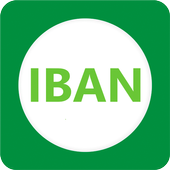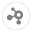Give us feedback# IBAN

Stable version 1.0.5 (Compatible with OutSystems 11)
5.0
(1 rating)# IBAN

Details
IBAN verification algorithm in C#

To check whether an IBAN is correct.

IBAN is described as follows in its Wikipedia entry:

The checksum is a basic ISO 7064 mod 97-10 calculation where the remainder must equal 1. To validate the checksum:

1. Check that the total IBAN length is correct as per the country. If not, the IBAN is invalid.
2. Move the four initial characters to the end of the string.
3. Replace each letter in the string with two digits, thereby expanding the string, where A=10, B=11, ..., Z=35.
4. Interpret the string as a decimal integer and compute the remainder of that number on division by 97.

The IBAN number can only be valid if the remainder is 1.

Release notes (1.0.5)

Improved IBAN length validation